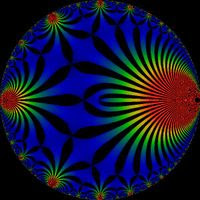## Modular Maypoles

Can anyone provide an update on Woit’s report on Witten’s new ideas? There seems to be no paper online, but one may materialise closer to Strings07.

From an M Theory point of view this $SO(2,2)$ theory represents a physical 2-Time domain, not merely a 2+1D model in a naive quantization scheme. Recall that for the $c=24$ case the partition function is exactly the function $J(q) = j(q) – 744$ where $j(q)$ is the famous q-expansion of the modular j-invariant (which we have been discussing) for $q = e^{2 \pi i \tau}$.

Now Matti Pitkanen has just been looking at how the Hurwitz zeta function (at the special point $a = 0.5$) naturally arises on imposing modular invariance on theta series. By definition, the j-invariant is invariant under modular transformations, so perhaps there is a simple relation between its theta function components and the components for the (let us call it) SUSY doublet of Hurwitz $\zeta (s,\frac{1}{2})$ and Riemann zeta functions. Starting with $\theta (0, \tau)$ we see that under $\tau \rightarrow \tau + 1$ this becomes $\theta_{01}(0, \tau)$. This in turn becomes $\theta (0, \tau)$ under a second transformation and both functions are part of the j-invariant triality. The third component $\theta_{10} (0, \tau)$ transforms to $(\sqrt{2}+ \sqrt{2} i) \theta_{10} (0, \tau)$ which is an 8th root of unity.This particular Hurwitz function is simply $\zeta_{H}(s) = (2^s – 1) \zeta (s)$, a simple multiple of the Riemann zeta function. According to Mathworld it has the interesting functional relation

$\zeta_{H}(s) = 2 (4 \pi)^{s-1} \Gamma (1-s) (sin \pi (1 + \frac{s}{2}). \zeta_{H}(1-s) + sin \pi (\frac{s}{2}). \zeta (1 – s))$

but this doesn’t seem quite right. This should be the usual functional relation for the Riemann zeta function. Anyway, the doublet $(\zeta, \zeta_H)$ describes the two term duality of the j-invariant.

## 8 Responses so far »

1. 1### Matti Pitkanen said,

Dear Kea.

there is something which I do not understand.

Let us look at the formulas for three thetas.

According to the first formula theta_(0,1) corresponds to theta (1/2,s) and thefore to Hurwitz zeta(s,1/2).

You however say that theta_(1,0) corresponds to zeta(s,1/2) which would thus be a simple function times zeta for which you give formula. Could you check whether there is confusion between index pairs [1,0] and [0,1]?

By the way, I realized an amusing connection with my much earlier work with elementary particle vacuum functionals, where theta functions with characteristic [a,b] for Riemann surface of genus g as function of Teichmueller parameters are the basic building blocks of modular invariant vacuum functionals defined in the finite-dimensional moduli space of partonic 2-surface characterizing the conformal equivalence class of the induced metric. Obviously, kind of spinorial variants of theta functions are in question with g+g spinor indices for genus g.

The recent case corresponds to g=1 Riemann surface (torus) so that a and b are g=1-component vectors having values 0 or 1/2. It seems more than obvious that the 4 theta functions must correspond to the Jacobi thetas. The values for a and b are 0 and 1 for them but this must be just a convention.

The extensions of modular group to fractional modular group that I discussed in my blog suggests the existence of (new kind of?, probably not) “quantum” theta functions with characteristics [a,b] with a and b being g-component vectors having fractional values k/n, k=0,1…n-1. This hierarchy would correspond to hierarchy of quantum groups for roots of unity and Jones inclusions and one could define also corresponding zeta function multiplets.

2. 2### Kea said,

Hi Matti. I agree that theta(1/2,s) is theta_{01}(s). The theta_{10} just appears as the extra bit of the j-invariant. I don’t think I used it elsewhere.

3. 3### Kea said,

OK, sorry, it isn’t clear that they are two separate paragraphs. And yeah, plenty of literature on q-thetas.

4. 4### Matti Pitkanen said,

Dear Kea,

suppose that fermions correspond to n=2 dark matter for which Z_2 would define conformal symmetry by M^4–>M^2/Z_2 and/or CP_2–>CP_2/Z_2 bundle structure. Quantum classical correspondence in strong form indeed requires a space-time correlate for spin 1/2 as n=2-fold covering.

If one assumes this one obtains only 3 fermion families since some theta functions with characteristic [a,b] always vanish for Riemann surfaces with genus g>2 and modular invariant vacuum functionals involve product of all thetas of this kind! No additional assumptions are needed. Hence it would seem that ordinary fermions are n=2 dark matter!

I would dare to guess that the fractionally modular invariant vacuum functionals for n>2 dark matter vanish also for g>2.

For more details see my posting.

5. 5### CarlBrannen said,

In the context of the fermions, 8th roots of unity aren’t as interesting as 12th roots of unity. Is there something that you could do to this that would make that change?

6. 6### Matti Pitkanen said,

Kea,

could you give reference to q-thetas. I would like to check whether they have anything to do with my proposal.

7. 7### Matti Pitkanen said,

If found a definition of in Wikipedia for 0<=|q| <1. This definition fails when q is root of unity which is the case of interest now. My proposal would extend the definition to the roots of unity but apply only in case of Riemann surfaces with Z_n conformal symmetry.

8. 8### Kea said,

Hi guys. Carl, the 8th roots are also interesting! Actually the theta functions appear to the power 3×8=24 in the j-invariant. Yes, Matti, I agree about the fermions, although our language is different. So, yet another way to count three generations. How many derivations do we need before people find this interesting?